# 用于多锂离子/锂聚合物电池充电器的集成解决方案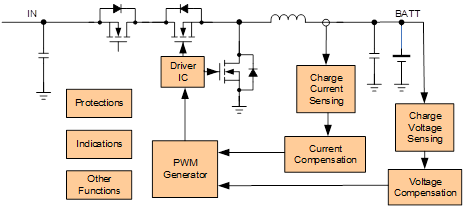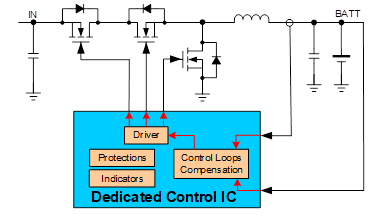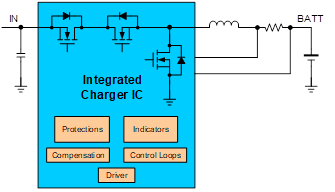组件 全集成 半集成 传统 充电器IC 1 0 0 功率MOSFET 0 3 3 电感 1 1 1 采样电阻 1 2 2 专用控制IC 0 1 0 驱动器 0 0 3 放大器和比较器 0 0 Several (5 to 10) MCU 0 0 1 大型组件总数量 3 7 15 to 20

## 全集成充电系统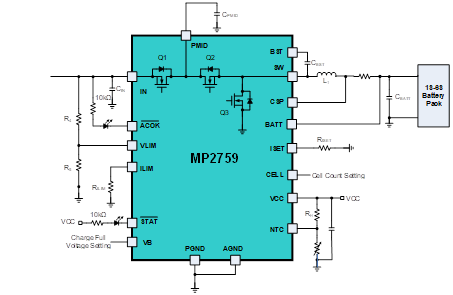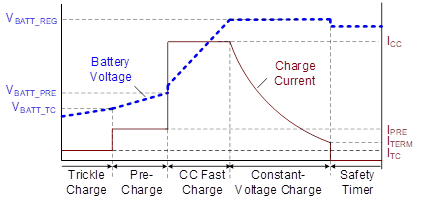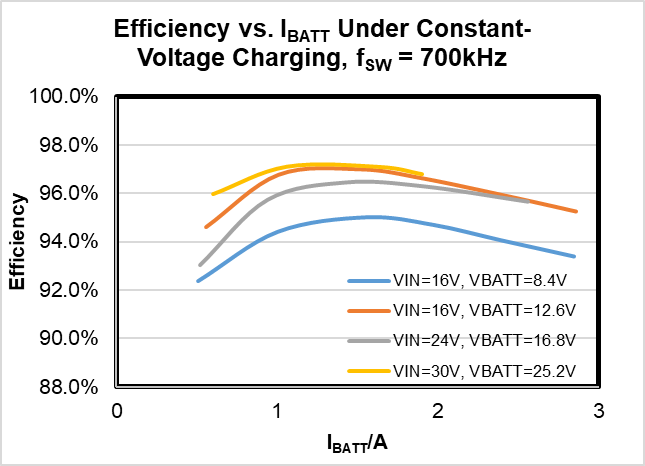((测试条件: L = 10µH/35mΩ, RSNS = 20mΩ)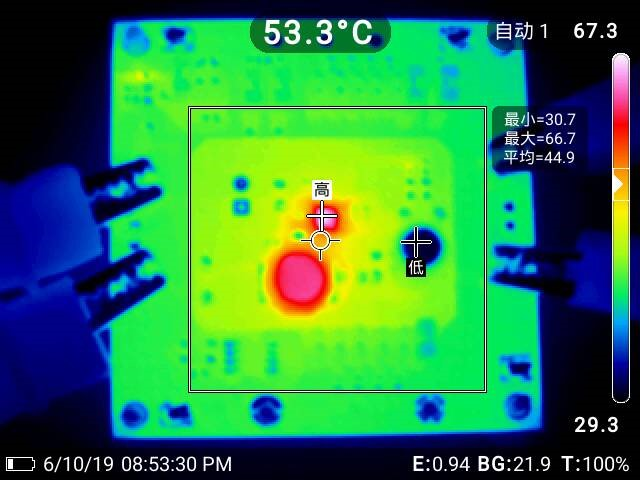(测试条件: VIN = 36V, VBATT = 24V, ICC = 2A, fSW = 700kHz, L = 10µH/35mΩ, RSNS = 20mΩ, 拷机时间20分钟)

(板信息: 63.5mmx63.5mm, 4层，2oz./层)

## 通过简单的外部电路实现功能扩展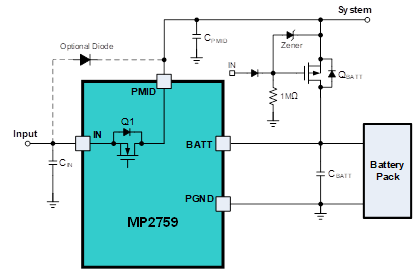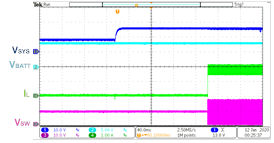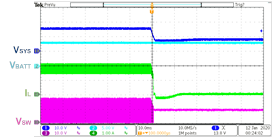(测试条件：VIN = 16V, VBATT = 8V, IIN_LMT = 2A, ICC = 3A, ILOAD = 1A)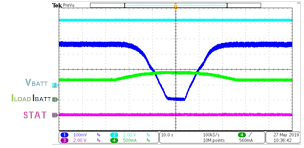(测试条件： VIN = 36V, VBATT = 8.48V, IIN_LMT = 1A, ICC = 2A)

## 通过MCU实时充电电流的微调

1. 以恒定电压读取流经电阻的电流；
2. 当电流保持恒定时，读取电阻上的电压。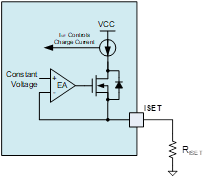$$I_{CHG} = \frac{R_{CONS}}{R_{ISET}}$$$$R_{EQ} = \frac {V_{CONS} R_1 G_{123}}{V_{CONS} G_{123} - (\frac {DUTY × V_{M\_PWM}}{R_2} + \frac {V_{CONS}} {R_1})}$$ $$G_{123} ={1 \over R_1} + { 1 \over R2} + {1 \over R_3}$$

$$R_{MAX\_ICHG} = R1 + R2 / R3$$

$$R_{1} = 0.5R_{MAX\_ICHG}$$

$$\biggl\{ \begin{array}{1} R_2//R_3 = R_{MAX\_ICHG} -R_1\\ \frac {MAX\_DUTY \space x \space V_{M\_PWM} - V_{CONS}}{R2} = \frac {V_{CONS}} {R_3} \end{array}$$

$$\frac {1}{2\pi (R_2//R_3)C_{ISET}}<< f_{PWM}$$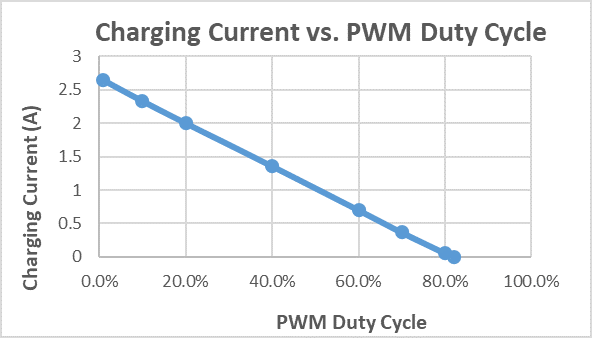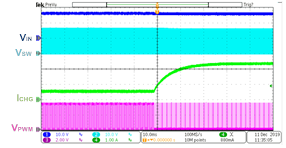a) PWM占空比从65%降至10%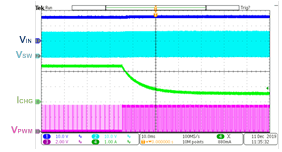b) PWM占空比从10%升至65%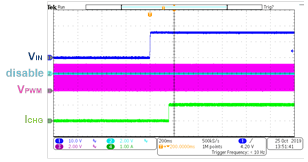a) VIN启动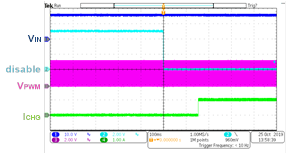b) 充电启用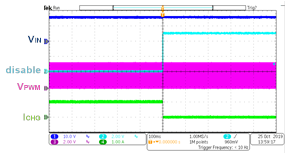c) 充电禁用

## 保护和指示功能

MP2759具有ACOK和STAT引脚，可用于指示输入源状态和IC操作状态。设计人员可以监视这些引脚上的信号，以确定设备是否正常充电。表2列出了不同输入源和工作条件下的ACOK和STAT状态。

 IN 充电状态 ACOK STAT 无 不适用 Hi-Z Hi-Z 有 充电中 低电平 低电平 有 充电完成、充电禁用 低电平 Hi-Z 有t NTC故障、安全定时器到期、电池过压保护（OVP） 低电平 以2Hz频率闪烁